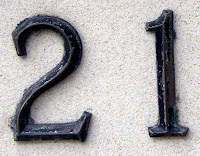## Monday, December 13, 2010

### 21

21 = 3 x 7.

21 is 10101 in base 2 (binary) and 111 in base 4.

21 has a unique representation as a sum of three squares: 21 = 12 + 22 + 42.

21 is the smallest number with five representations as a sum of three primes: 21 = 2 + 2 + 17 = 3 + 5 + 13 = 3 + 7 + 11 = 5 + 5 + 11 = 7 + 7 + 7.

21 is the eighth Fibonacci number.

21 is the fifth Motzkin number.

21 is the sixth triangular number.21 is the total number of spots on a normal die.

Source: Phillips, R. 2004. Numbers: Facts, Figures and Fiction. Badsey Publications.